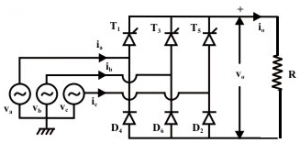Home > ac dc power converters > three phase half controlled rectifier

Three phase Half controlled rectifier

Three phase converters
Three phase converters provide higher average output voltage.
Frequency of ripples on output voltage is higher compared with that of single phase converter. Thus the filtering requirements for smoothing out load current & load voltage are simpler. For these reasons, three phase converters are extensively used in high power variable speed drivers.

Three phase semiconverters

Three single phase half wave converters can be connected to form a three phase half wave converter. Similarly three phase semiconverter uses 3 SCRs T1, T3 & T5 and 3 diodes D2, D4& D6
R,Y,B are phase voltages with respect to 'N'.In the circuit shown above when any device conducts,line voltage is applied across load. so line voltage are necessary to draw
Phase shift between two line voltages is 60 degree & between two phase voltages it is 120 degree Each phase & line voltage is sine wave with the frequency of 50 Hz.

Operation of semiconverters with R load
With purely resistive load current will be in phase with load voltage.
Depending on value of firing angle, circuit can operate in two different modes.
Mode1- when α<60
T1 is triggered at α=30. SCR T1 and D6 conduct and line voltage RY is applied to the load from (π/6 +α) to π/2. 60. At π/2 'D2' is more positive so conducts. Therefore, line voltage RB is applied to load. This continues up to firing of T3 at π/6 +α
Vo(avg)=(3√3Vm)/2π(1+cosα)
For α≤60 the output voltage is continuous so called continuous mode of operation.
For α> 60 for some duration of time no device conducts so called discontinuous mode of operation.